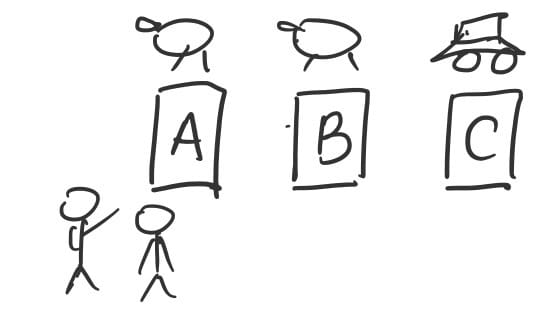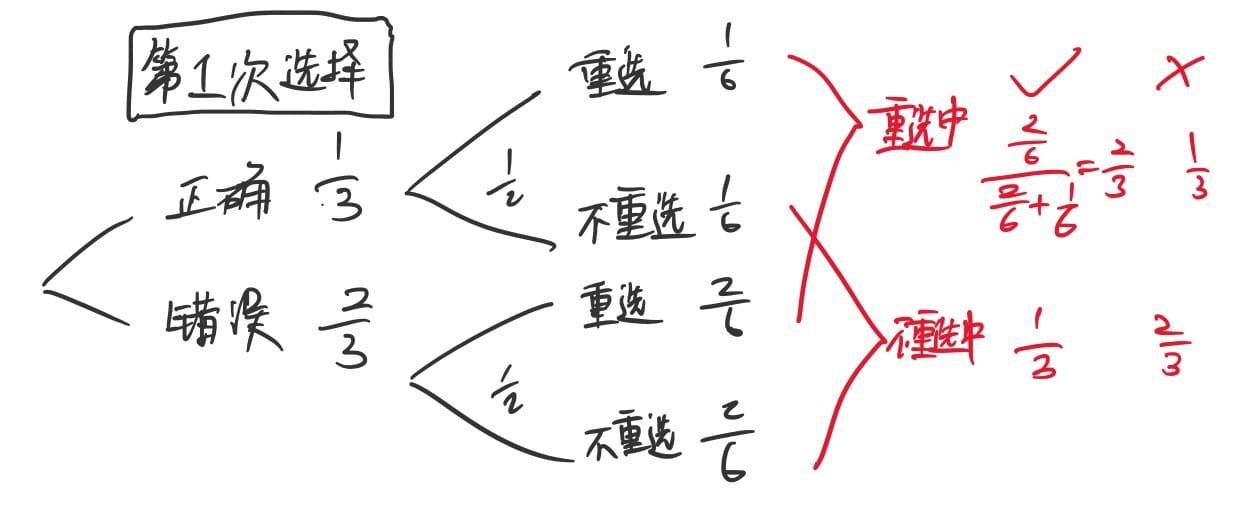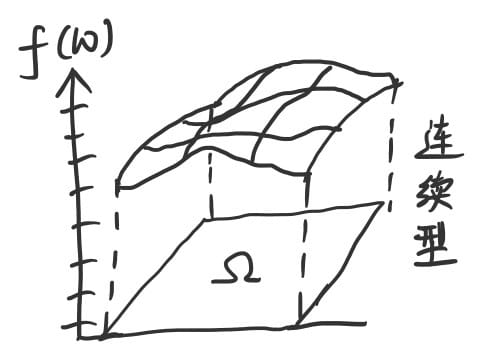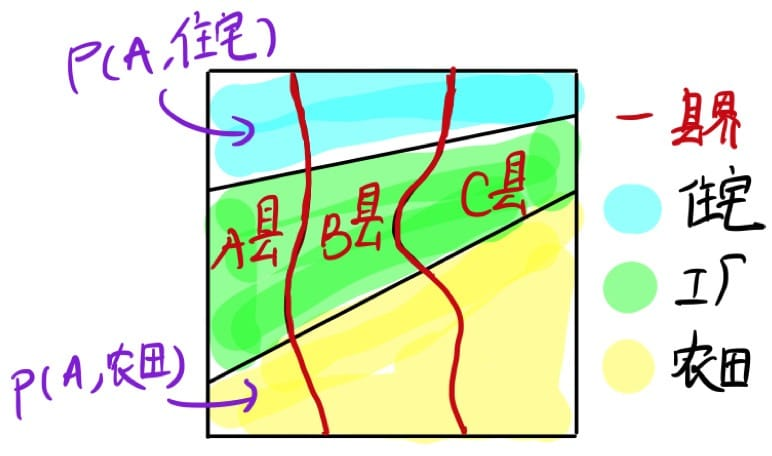## 概率的定义

新的视角看待问题不是一件令人激动的事吗？概率是面积（测度）！

### 蒙提霍尔问题（Monty Hall Problem）• 如果第一次选择正确，重选必定错误（$\frac{1}{3}$）
• 如果第一次选择错误，重选必定正确（$\frac{2}{3}$ 即第一次选错的概率即为「重选后正确」的概率）1. 问题中要考虑主持人已打开错误的门的条件（prior）

2. 如果挑战者第一次就选错了，主持人会不会打开一扇门让他再选呢？（肯定会！仔细想一下）

3. 考虑有 100 扇门而主持人将打开其中 98 扇的情况（马住）

4. 上帝视角观看所有情况将不确定的事件（抽象的概率、生成机制）转换为确定的事件（统计观测值）

• 每个会场同时进行游戏
• 每个会场按照事先确定好的剧本进行游戏（确定事件）
• 另一个角度，每个会场即为问题的一个平行世界 $\omega$ 且 $\omega \in \Omega$（下面有对三元组的解释）
⭕️：跑车 ❌：山羊 挑战者选择 A 门 挑战者选择 B 门 挑战者选择 C 门

### 三元组和术语

$$(\Omega,\mathcal{F},P)$$

#### 随机变量## 随机变量之间的关系

### 问题引入$\Omega$ 国有 3 个县（A、B、C），面积分别为 $P(A)$、$P(B)$、$P(C)$，且整个国家的总面积为 $1$。

$$P(A)+P(B)+P(C)=1$$

$$P(住宅)+P(工厂)+P(农田)=1$$

### 概念的预热

• 联合概率：面积 $P(住宅,用途)$
• 边缘概率：面积 $P(住宅=A)$（不考虑某个值的变化）
• 条件概率：特定县、特定用途的比例（条件概率就是一个比例）
• 贝叶斯公式：倒推比例并理解其含义
• 独立性：比例相同的情况（如下）
• $P(住宅|A)=P(住宅|B)=P(住宅|C)=P(住宅)$
• $P(住宅,A)=P(A)P(住宅|A)=P(A)P(住宅)$

### 分解联合概率（从右往左读更容易理解【面积 + 比例】）：

$P(AA, BB, CC) = P(AA | BB, CC)P(BB, CC) = P(AA | BB, CC)P(BB | CC)P(CC)$

$P(AA,BB|CC)=P(AA|BB,CC)P(BB,CC)$（左右乘以 $P(CC)$ 后和上一条式子一样）

$P(A, B, C, D) = P(A, B | C, D)P(C|D)P(D)$

### 用上面的概念解决三扇门问题

\begin{aligned} &P(X=3|Y=3,Z=1) = \frac{P(X=3,Y=3,Z=1)}{P(Y=3,Z=1)}\ &= \frac{P(X=3,Y=3,Z=1)}{P(X=1,Y=3,Z=1)+P(X=2,Y=3,Z=1)+P(X=3,Y=3,Z=1)} \end{aligned}

$$P(Y=1)=P(Y=2)=P(Y=3)=\frac{1}{3}$$

$$P(Y=3|X=1)=P(Y=3|X=2)=P(Y=3|X=3)=\frac{1}{3}$$

$P(Z=1|X=1, Y=3)=0$ …… 不可能打开正确的门
$P(Z=1|X=2, Y=3)=1$ …… 另一扇门是正确答案，因此只能打开门 $1$
$P(Z=1|X=3, Y=3)=\frac{1}{2}$ …… 剩下的两扇门都不是正确答案，因此概率为 $\frac{1}{2}$

$P(X=1,Y=3,Z=1) = P(Z=1|X=1,Y=3)P(Y=3|X=1)P(X=1)=0$
$P(X=2,Y=3,Z=1) = \frac{1}{9}$（同理）
$P(X=3,Y=3,Z=1)= \frac{1}{18}$（同理）

### 贝叶斯公式

• 通信：根据含有噪声的接收信号 Y 推测发送信号 X
• 语音识别：根据麦克风识别的音频波形数据 Y 推测语音信息 X
• 文字识别：根据扫描仪读取的图像数据 Y 推测用户书写的问童子 X
• 邮件自动过滤：根据收到的邮件文本 Y 推测邮件的类型 X（是否为广告等）

• 已知所有的：P(原因) 与 P(结果|原因)
• 求：P(原因|结果)

### 独立性

「独立」与「互斥」不同（重要❗️）

$$P(发现陷阱 | 有陷阱) = P(发现陷阱 | 没有陷阱)$$

$$or$$

$$P(有陷阱|发现陷阱)= P(有陷阱|没发现陷阱)$$

1. 我们无需区分谁独立于谁，没有先后关系。
2. 上面第一条式子中，当 $P(有陷阱) = 0 或 1$ 时，虽然没有意义，但独立性仍然成立。
3. $P(发现陷阱|有陷阱)=P(没发现陷阱|有陷阱)$ 并不能判断两者是否独立。

（1）「这张牌是黑桃」与「这张牌是人头牌」是否独立？
（2）「这张牌是黑桃」与「这张牌是红桃」是否独立？

1. ⭕️ 与 ❌ 独立

2. 条件概率与条件无关【直观易懂】

$P(⭕️|❌) = P(⭕️|¬❌)$

3. 添加或去除条件不影响概率【直观易懂】

$P(⭕️|❌) = P(⭕️)$

4. 联合概率之比相同

$P(⭕️,❌):P(⭕️,¬❌) = P(¬⭕️,❌) = P(¬⭕️,¬❌)$

5. 联合概率是边缘概率的乘积【适用性最广，便于计算和判断，满足上面条件无意义的情况】

$P(⭕️,❌)=P(⭕️)P(❌)$【可通过贝叶斯公式推导】

「定义」与「定理」是完全不同的概念。「定义」是某样事物的固定名称。在数学中，我们无需解释某一定义背后的理由。尽管该定义的产生必然有其原因，但数学并不关心定义者的动机。另一方面，「定理」则是由前提推导出的结论。数学需要严格证明该推导过程的正确性。

Y = ❌ Y = ¬❌
X = ⭕️ 0.10 0.30
X = ¬⭕️ 0.15 0.45

1. $P(A_1, A_2, …, A_n)= P(A_1)P(A_2)\cdots P(A_n)$
2. 任意 n - 1 个事件都相互独立

\begin{aligned} P(A,B,C)&=P(A)P(B)P(C)\ P(A,B)&=P(A)P(B)\ P(A,C)&=P(A)P(C)\ P(B,C)&=P(B)P(C) \end{aligned}